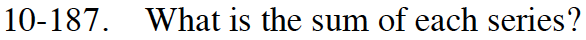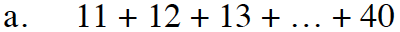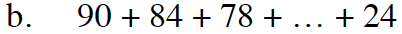### Home > INT3 > Chapter 10 > Lesson 10.3.3 > Problem10-187

10-187.
1. What is the sum of each series? Homework Help ✎

1. 11 + 12 + 13 + … + 40

2. 90 + 84 + 78 + … + 24Find the sequence that describes the series.

t(n) = 10 + n

Find which term is 40.

(40) = 10 + n
30 = n

Find the sum using the formula

$S(k)=\frac{k(t(1)+t(k))}{2}$

$S(30)=\frac{30(11+40)}{2}$See part (a).